# Pipe Flow Wizard Software - Size Diameter Calculator

The Pipe Flow Wizard software will calculate the minimum internal pipe diameter that is required to achieve a given flow rate for a specified maximum pressure loss. The software will consider the effect of pipe friction losses, taking account of the pipe material, internal roughness of the pipe, the length of pipe, the fluid flow rate, the maximum allowed pressure drop, the fluid density, the fluid viscosity, and the pressure drop through any fittings and bends.

## Liquids and Gases

The Pipe Flow Wizard software uses accurate Colebrook-White friction factors with the Darcy Weisbach equation when calculating friction loss and overall pressure loss in the pipe for liquids.

Pipe Flow Wizard uses Compressible Isothermal Flow equations when calculating the friction loss and total pressure loss in the pipe for gas flow. The software supports different compressible flow equations, including the General Fundamental Flow equation, Complete Isothermal Flow equation, AGA Flow equation, Weymouth Flow equation, Panhandle A Flow equation, Panhandle B Flow equation, and the IGT Flow equation.

## Pipes and Fittings

The Pipe Flow Wizard software allows the user to easily select from standard data (which can be added to) for pipe material, pipe diameter, pipe fittings and the fluid properties to be used in the calculation.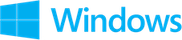Find Diameter calculation and results screenshots from Pipe Flow Wizard Software for Windows.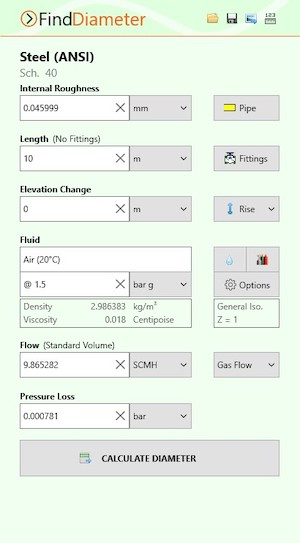Find Diameter Calculation Screen (Windows)Find Diameter Results Screen (Windows)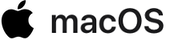Find Diameter calculation and results screenshots from Pipe Flow Wizard Software for macOS.Find Diameter Calculation Screen (macOS)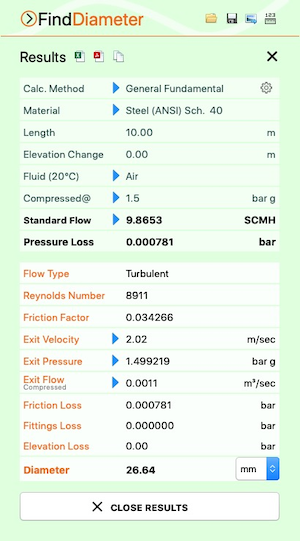Find Diameter Results Screen (macOS)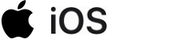Find Diameter calculation and results screenshots from Pipe Flow Wizard Software for iOS.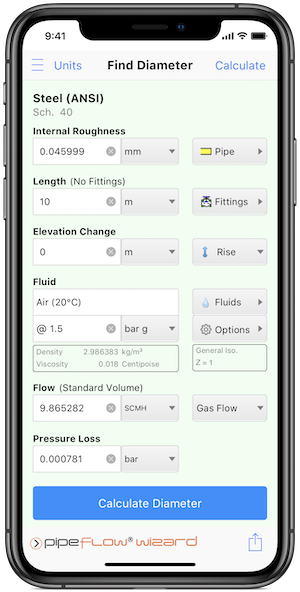Find Diameter Calculation Screen (iOS)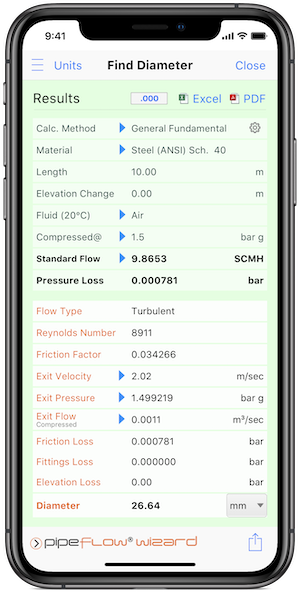Find Diameter Results Screen (iOS)

Secure Online PaymentsPipe Flow Software: Piping design, Pressure drop calculator, Flow rate calculator, Pump head calculations, Pump selection software.  Copyright © Pipe Flow Software 1997-2022